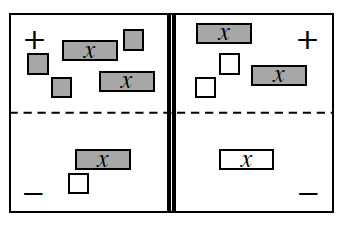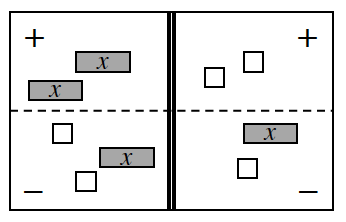### Home > CC3 > Chapter 3 > Lesson 3.1.2 > Problem3-14

3-14.

Write the equation represented in each diagram below on your paper. For each part, simplify as much as possible and then solve for $x$. Be sure to record your work on your paper.1. 3-14a HW eTool (CPM)Write the original equation.

Remove zero pairs.

Combine like terms.

Remove an $x$-tile from both sides.

Add $2$ to both sides.

Divide by $2$ on both sides.

$2x+3-(x-1)=2x-2-(-x)$

$2x+3+(−x)+1=2x+(−2)+x$

$2x+(−x)+3+1=2x+x+(−2)$

$x+3+1=2x+x+(−2)$

$x+4=3x−2$

$4=2x−2$

$6=2x$

$3=x$

1. 3-14b HW eTool (CPM)Follow steps similar to part (a).

Use the eTools below to help solve the problems.
Click the link at right for the full version of the eTool: 3-14a HW eTool (CPM)

Click the link at right for the full version of the eTool: 3-14b HW eTool (CPM)